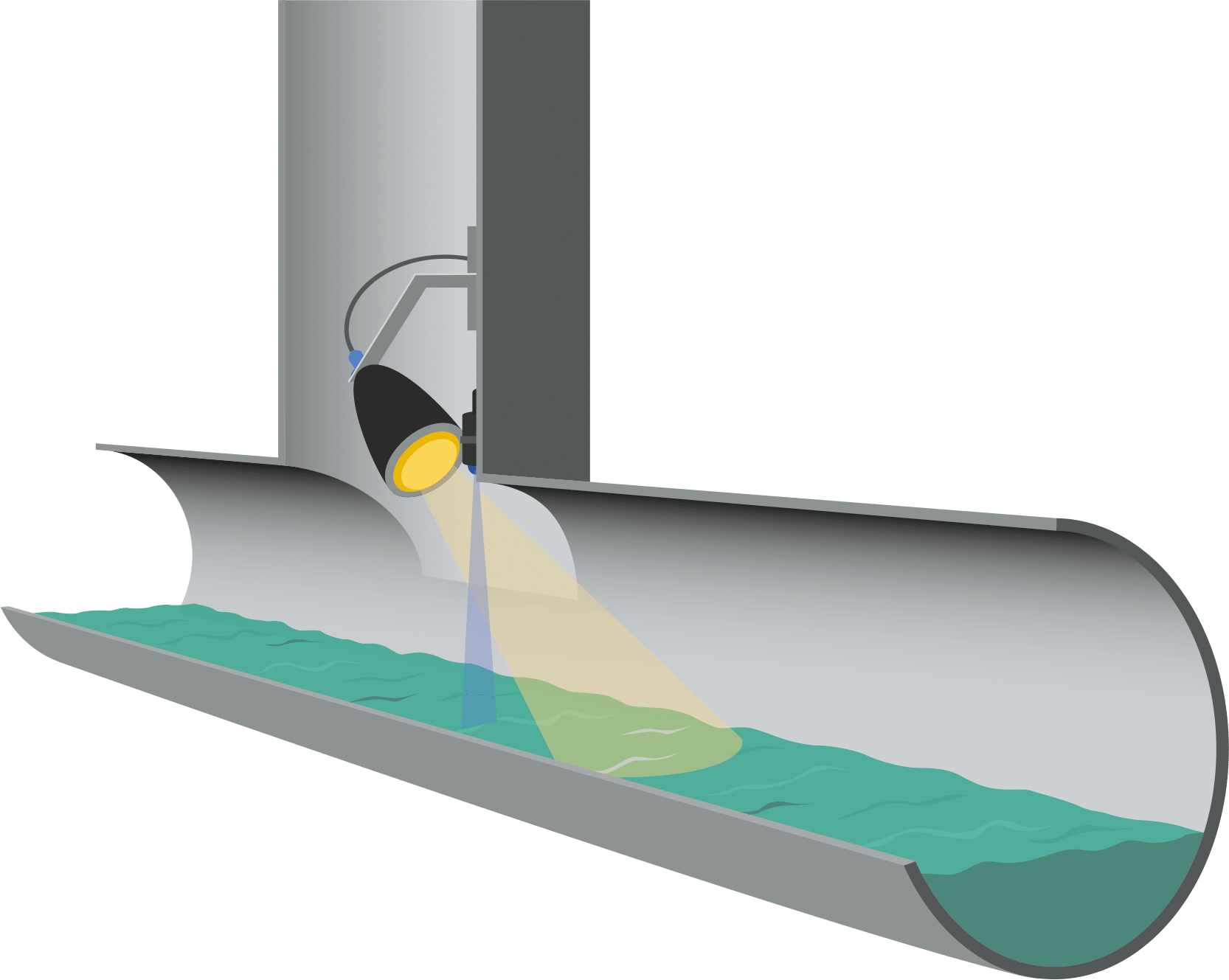# How To Calculate Sonic Velocity

In this post I want to share how to calculate sonic velocity. Before we jump into the equation, let’s review about sonic velocity briefly.

Sonic velocity or critical velocity is the maximum velocity that can be attained by a compressible fluid. We usually use this parameter in sizing pipe, control valve, and flare.

Sonic velocity is a function the following parameters:

• Ratio of specific heats in the gas (k or Cp/Cv)
• Temperature
• Molecular weight of the gas

The molecular weight is expressed in absolute temperature, therefore we will use temperature unit of oR or oK.

Then how to calculate?

If we use imperial units, then use the following equation to calculate sonic velocity:

Vs (sonic velocity) = 223 (k T/M)0.5

Where:

Vs = sonic velocity (ft/s)

k = ratio of specific heats in the gas (Cp/Cv)

T = absolute temperature (oR)

M = molecular weight of gas

If we use metric units, then use the following equation to calculate sonic velocity:

Vs (sonic velocity) = 91.2 (k T/M)0.5

Where:

Vs = sonic velocity (m/s)

k = ratio of specific heats in the gas (Cp/Cv)

T = absolute temperature (oK)

M = molecular weight of gas